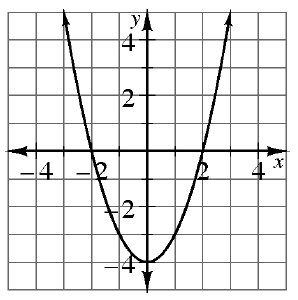### Home > INT2 > Chapter 11 > Lesson 11.2.1 > Problem11-49

11-49.

Examine the graph of $y = f(x)$ at right. Use the graph to determine the following values.

The value $f(x)$ is equal to the value y obtained by substituting a number $x$ into the function.

1. $f(1)$

$-3$

1. $f(0)$

If $x = 0$,
then $y =$ ?

1. $x$ if $f(x) = 4$

$≈ 2.8$ and $-2.8$

1. $x$ if $f(x) = 0$

If $y = 0$,
then $x =$ ?